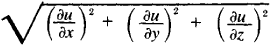(redirected from Gradient of a scalar)
Also found in: Dictionary, Thesaurus, Medical, Financial.

1. a part of a railway, road, etc., that slopes upwards or downwards; inclination
2. a measure of such a slope, esp the ratio of the vertical distance between two points on the slope to the horizontal distance between them
3. Physics a measure of the change of some physical quantity, such as temperature or electric potential, over a specified distance
4. Maths
a. (of a curve) the slope of the tangent at any point on a curve with respect to the horizontal axis
b. (of a function, f(x, y, z)) the vector whose components along the axes are the partial derivatives of the function with respect to each variable, and whose direction is that in which the derivative of the function has its maximum value. Usually written: grad f, ∇f or ∇f

The slant of a road, piping, or the ground; expressed as a percentage of slope from true horizontal.

in biology, a regular quantitative change in morphological or functional (including biochemical) properties along one of the axes of the body (or organ) at any stage of its development.

Examples of gradient include the diminution of yolk content in the eggs of amphibians in a direction from the vegetal pole to the animal pole and the varying sensitivity to poisons and dyes of different sections of the bodies of coelenterates and worms. A gradient that reflects decrease or increase in the rate of metabolism or other physiological indexes is called a physiological or metabolic gradient. An example in vertebrates of physiological gradient is the decrease in the capacity for automatic contraction in areas of the heart from the venous end to the aortal. The location of the highest manifestation of function is called the highest level of the gradient; the section with the lowest manifestation of function is called the lowest level of the gradient. According to the American scientist C. Child, physiological gradient is the primary cause of differentiation in the embryo and of integration in the adult organism; however, gradient frequently is not the cause but only the consequence of broader biological principles of development.

L. V. BELOUSOV

a vector indicating the direction of maximum change of some quantity, the value of which varies from point to point in space. If the quantity is expressed as the function u(x, y, z), then the components of the gradient are ∂u/∂x, ∂u/∂y, ∂u/∂z; gradient is denoted by the symbol grad u. At any point the gradient is normal to the constant-value surface of the function u(x, y, z) at that point and its magnitude isThe concept of gradient is widely used in physics, meteorology, oceanography, and other sciences to indicate the space rate of change of some quantity when shifting for the unit length in the direction of the gradient; for example, pressure gradient, temperature gradient, humidity gradient, gradient of wind velocity, gradient of salinity, gradient of ocean water density, and gradient of electrical potential, better known as electric field intensity.

[′grād·ē·ənt]
(geology)
The rate of descent or ascent (steepness of slope) of any topographic feature, such as streams or hillsides.
(mathematics)
A vector obtained from a real function ƒ(x1, x2,…, xn ) whose components are the partial derivatives of ƒ; this measures the maximum rate of change of ƒ in a given direction.
A slope expressed in feet per mile as a ratio of the horizontal to the vertical distance; for example, 40:1 means 40 feet horizontally to 1 foot vertically.

A GradientThe software generates the gradient automatically as in this example created in Photoshop.